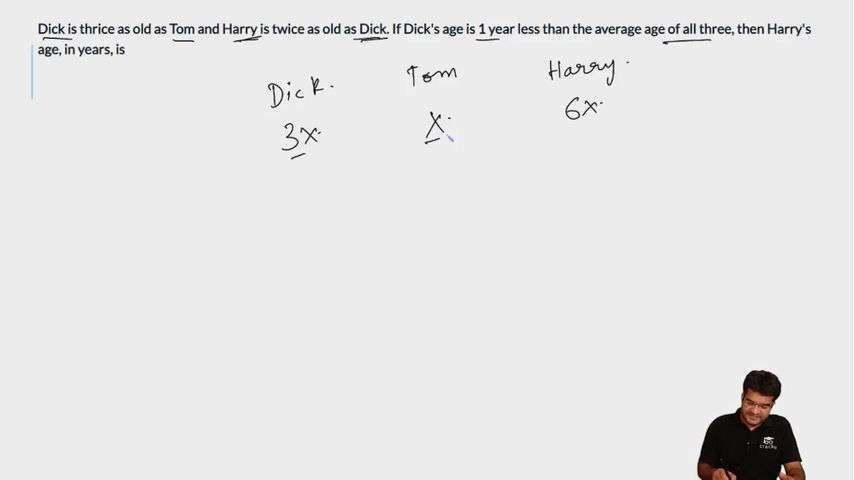Question 57

# Dick is thrice as old as Tom and Harry is twice as old as Dick. If Dick's age is 1 year less than the average age of all three, then Harry's age, in years, is

Solution

Let tom's age = x

=> Dick=3x

=>harry = 6x

Given,

3x+1 = (x+3x+6x)/3

=> x= 3

Hence, Harry's age = 18 years

### View Video Solution